Best for live in-class or video conferencing lessonsStart teacher-led lesson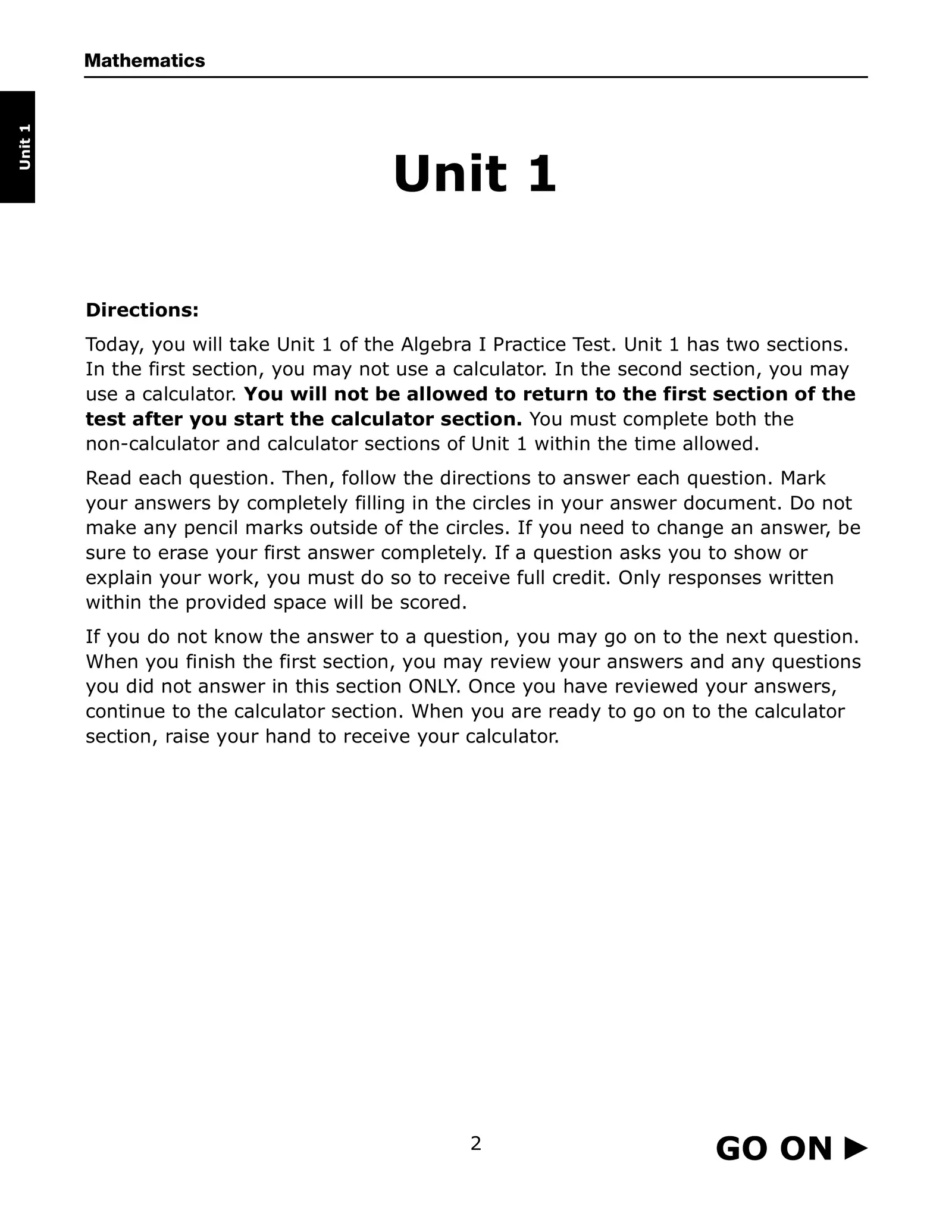Mathematics Unit 1 GO ON TO NEXT PAGE 4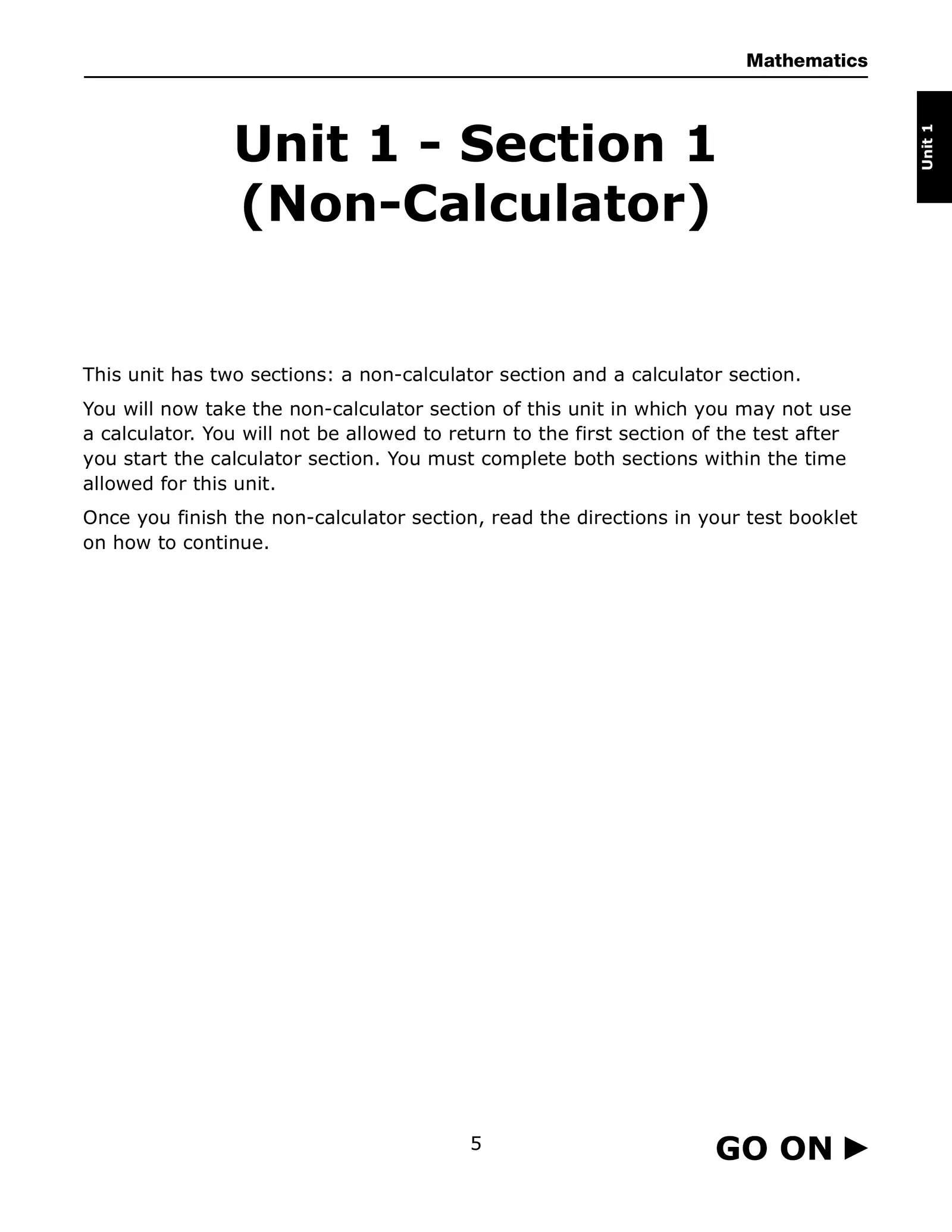Mathematics Unit 1 Unit 1 - Section 1 (Non-Calculator) This unit has two sections: a non-calculator section and a calculator section. You will now take the non-calculator section of this unit in which you may not use a calculator. You will not be allowed to return to the first section of the test after you start the calculator section. You must complete both sections within the time allowed for this unit. Once you finish the non-calculator section, read the directions in your test booklet on how to continue. 5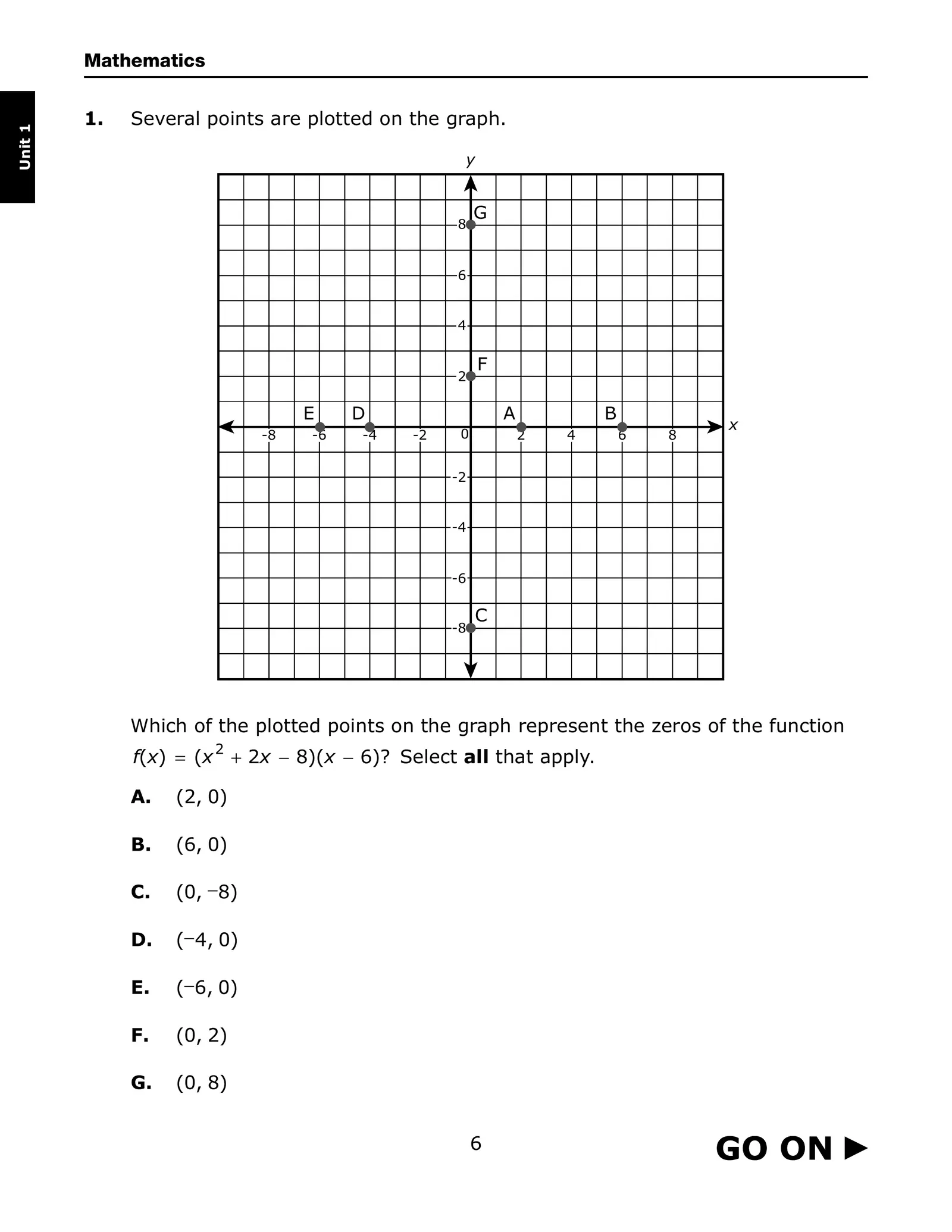Mathematics 1. Several points are plotted on the graph. Unit 1 y G 8 6 4 F 2 E D A B x -8 -6 -4 -2 0 2 4 6 8 -2 -4 -6 C -8 Which of the plotted points on the graph represent the zeros of the function f(x) = (x 2 + 2x − 8)(x − 6)? Select all that apply. A. (2, 0) B. (6, 0) C. (0, −8) D. (− 4, 0) E. (−6, 0) F. (0, 2) G. (0, 8) 6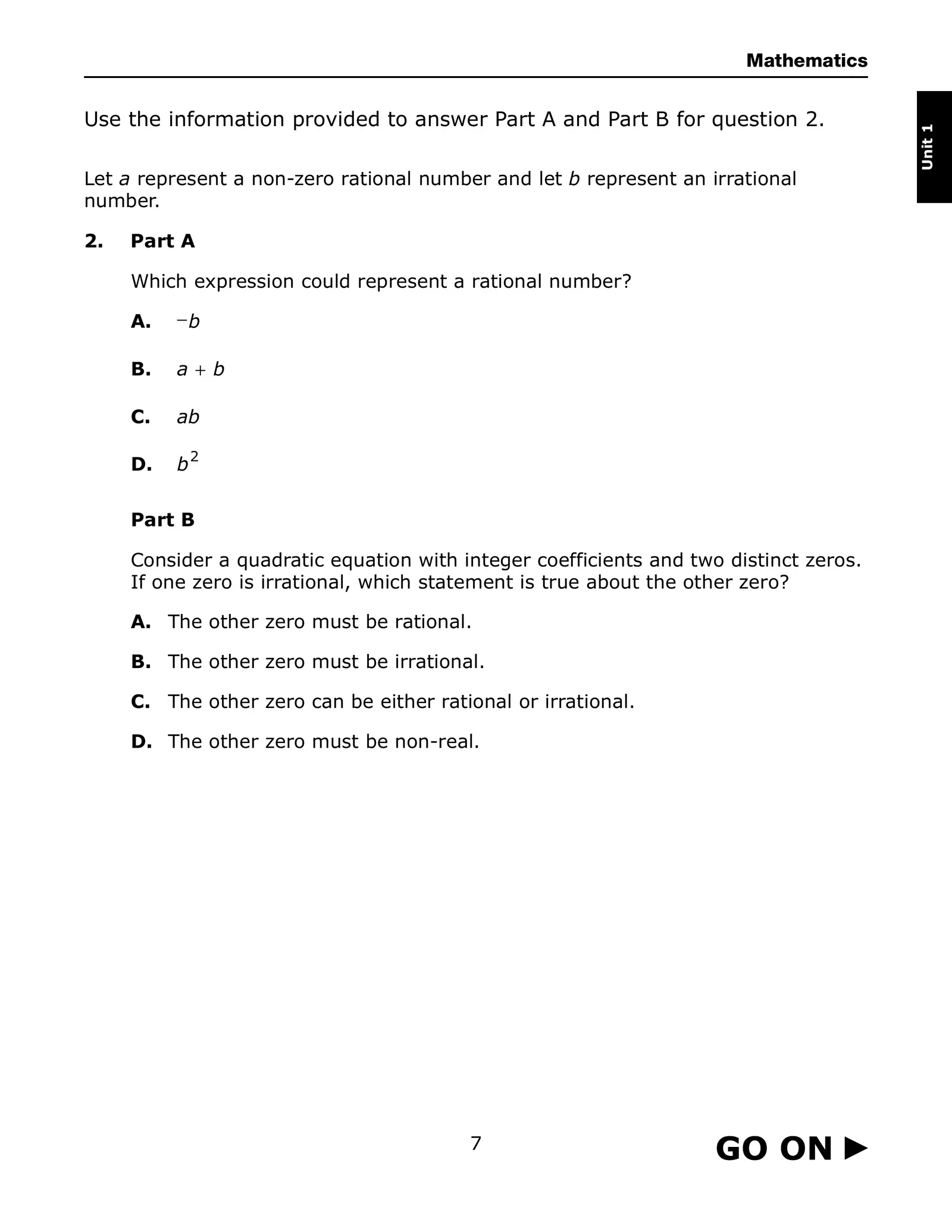Mathematics Use the information provided to answer Part A and Part B for question 2. Unit 1 Let a represent a non-zero rational number and let b represent an irrational number. 2. Part A Which expression could represent a rational number? A. −b B. a+ b C. ab D. b2 Part B Consider a quadratic equation with integer coefficients and two distinct zeros. If one zero is irrational, which statement is true about the other zero? A. The other zero must be rational. B. The other zero must be irrational. C. The other zero can be either rational or irrational. D. The other zero must be non-real. 7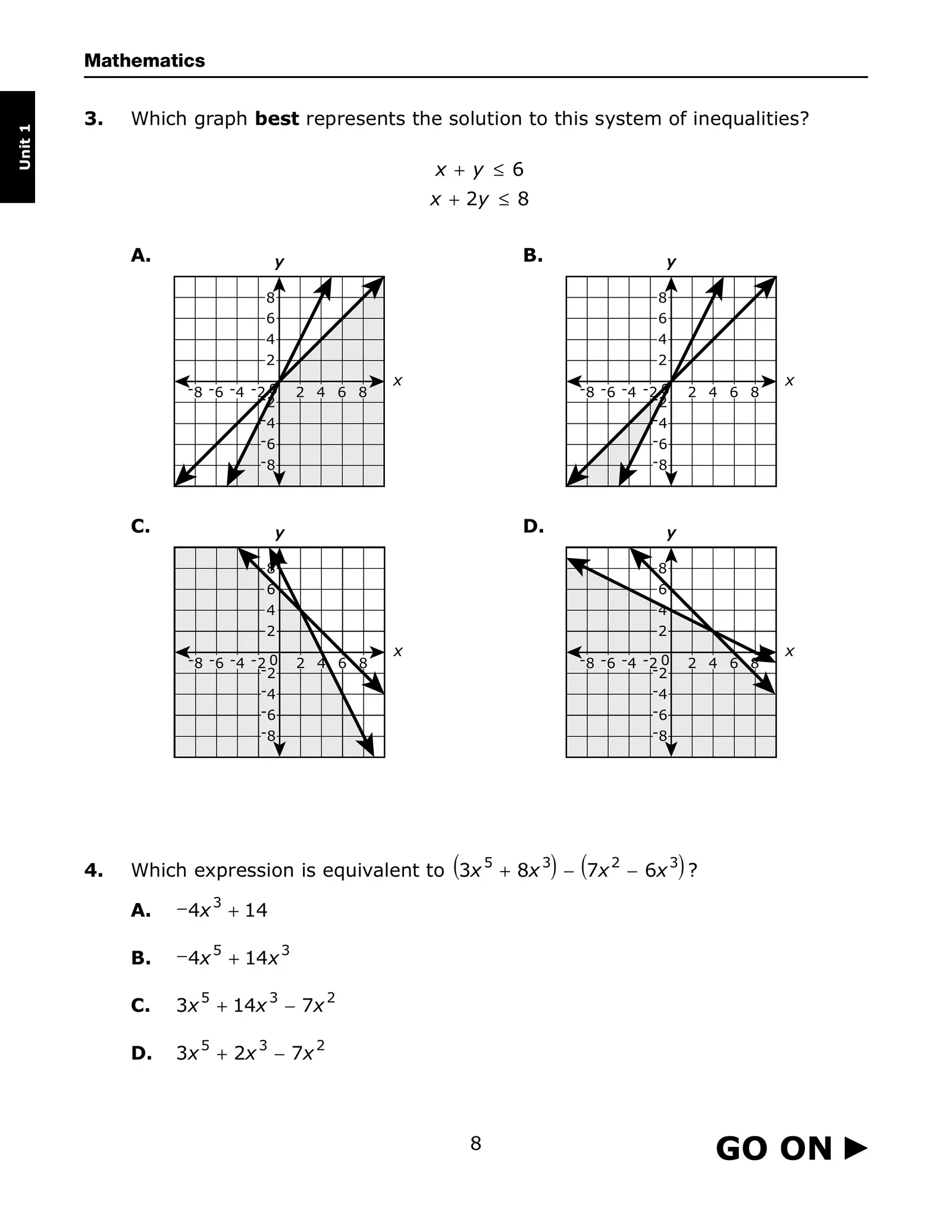Mathematics 3. Which graph best represents the solution to this system of inequalities? Unit 1 x + y ≤ 6 x + 2y ≤ 8 A. y B. y 8 8 6 6 4 4 2 2 x x -8 -6 -4 -2 0 2 4 6 8 -8 -6 -4 -2 0 2 4 6 8 -2 -2 -4 -4 -6 -6 -8 -8 C. y D. y 8 8 6 6 4 4 2 2 x x -8 -6 -4 -2 0 2 4 6 8 -8 -6 -4 -2 0 2 4 6 8 -2 -2 -4 -4 -6 -6 -8 -8 4. ( ) ( Which expression is equivalent to 3x 5 + 8x 3 − 7x 2 − 6x 3 ? ) A. − 4x 3 + 14 B. − 4x 5 + 14x 3 C. 3x 5 + 14x 3 − 7x 2 D. 3x 5 + 2x 3 − 7x 2 8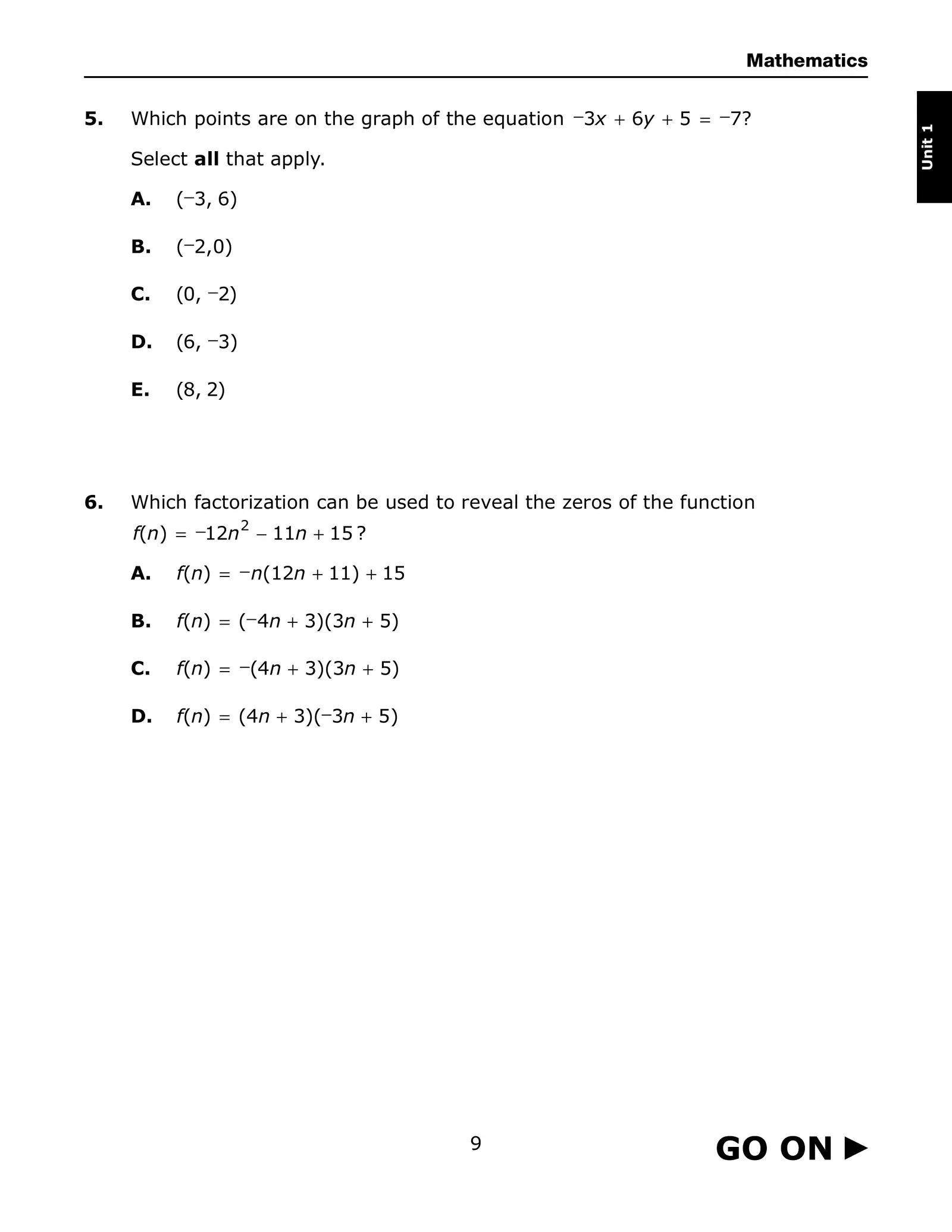Mathematics 5. Which points are on the graph of the equation −3x + 6y + 5 = −7? Unit 1 Select all that apply. A. (−3, 6) B. (−2,0) C. (0, −2) D. (6, −3) E. (8, 2) 6. Which factorization can be used to reveal the zeros of the function f(n) = −12n 2 − 11n + 15 ? A. f(n) = − n(12n + 11) + 15 B. f(n) = (− 4n + 3)(3n + 5) C. f(n) = −(4n + 3)(3n + 5) D. f(n) = (4n + 3)(−3n + 5) 9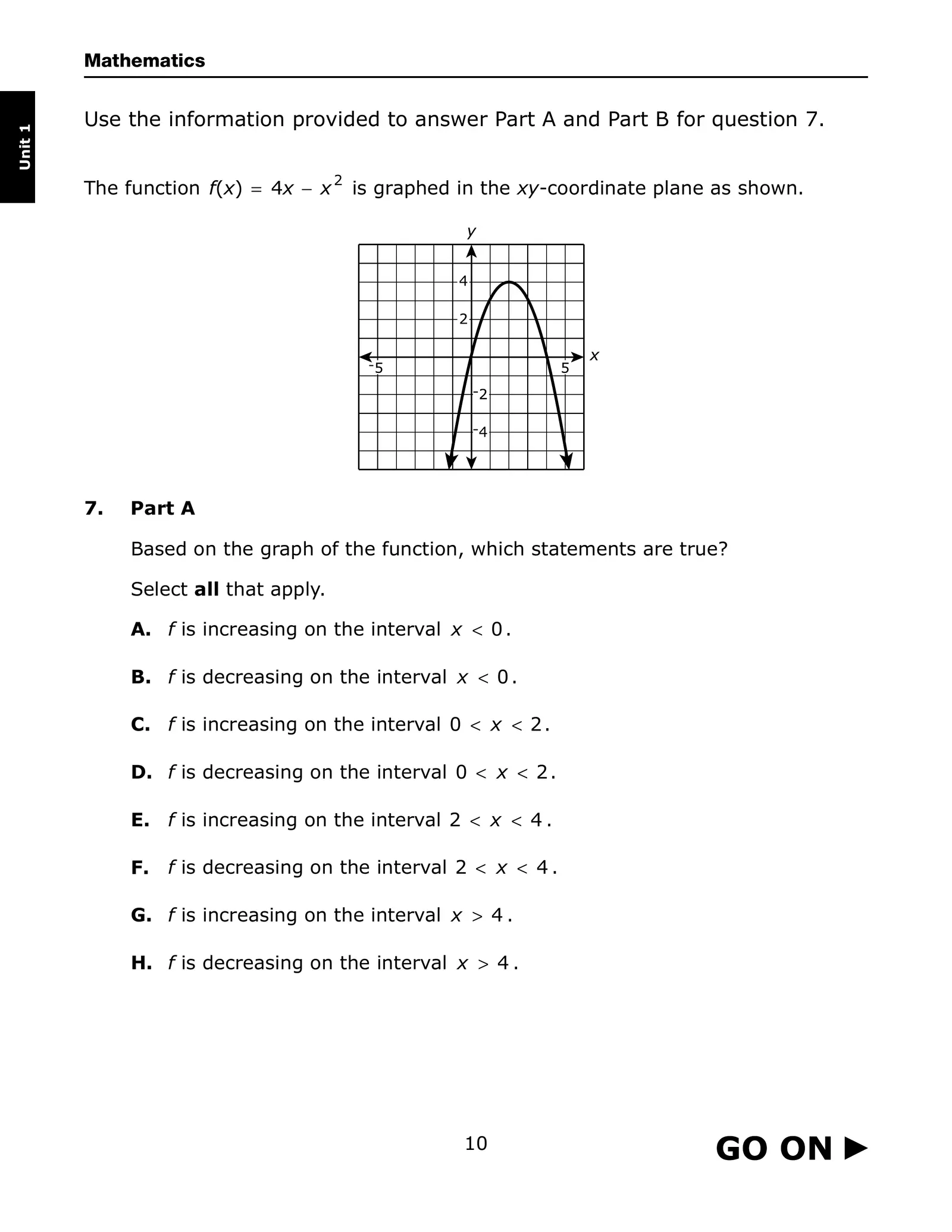Mathematics Use the information provided to answer Part A and Part B for question 7. Unit 1 2 The function f(x) = 4x − x is graphed in the xy-coordinate plane as shown. 7. Part A Based on the graph of the function, which statements are true? Select all that apply. A. f is increasing on the interval x < 0 . B. f is decreasing on the interval x < 0 . C. f is increasing on the interval 0 < x < 2 . D. f is decreasing on the interval 0 < x < 2 . E. f is increasing on the interval 2 < x < 4 . F. f is decreasing on the interval 2 < x < 4 . G. f is increasing on the interval x > 4 . H. f is decreasing on the interval x > 4 . 10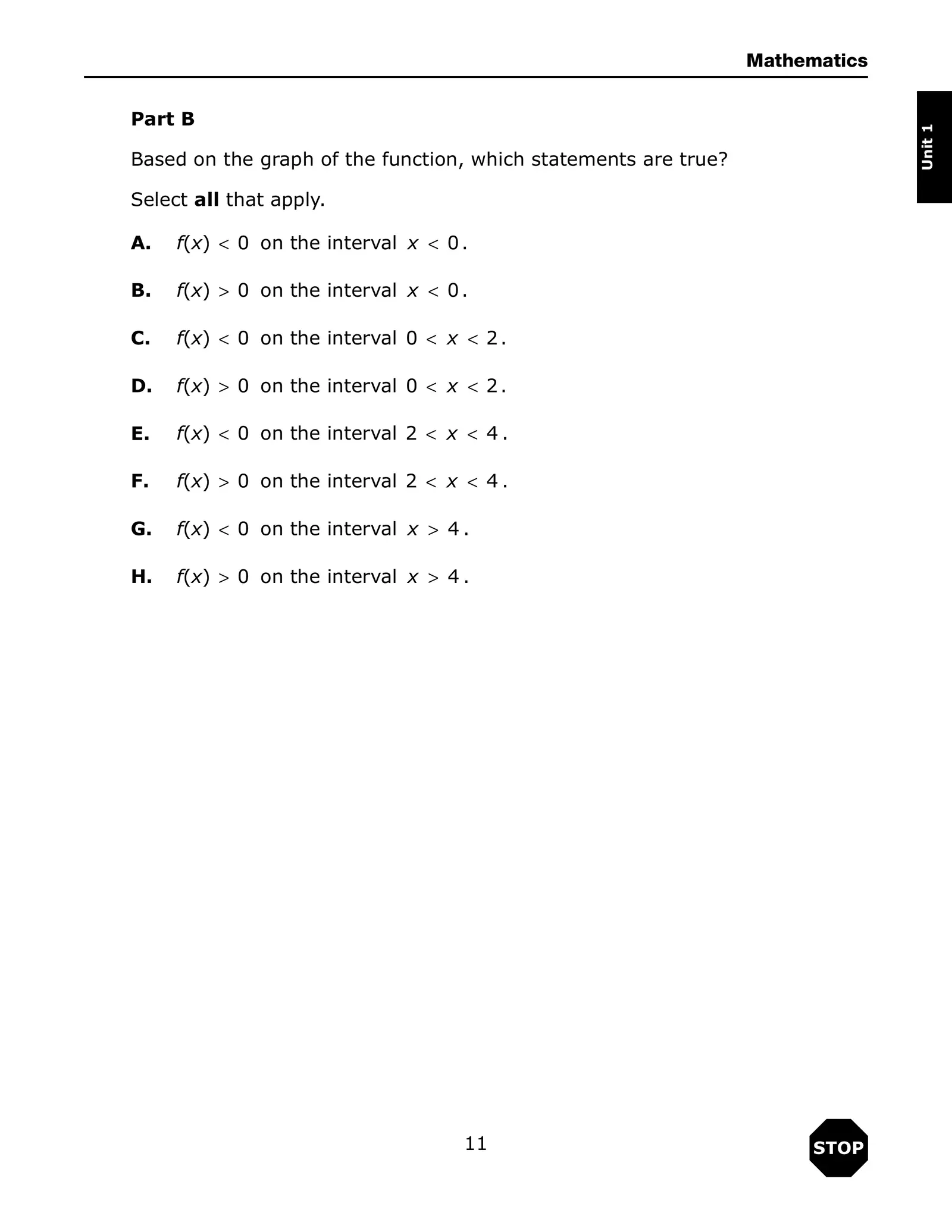Mathematics Part B Unit 1 Based on the graph of the function, which statements are true? Select all that apply. A. f(x) < 0 on the interval x < 0 . B. f(x) > 0 on the interval x < 0 . C. f(x) < 0 on the interval 0 < x < 2 . D. f(x) > 0 on the interval 0 < x < 2 . E. f(x) < 0 on the interval 2 < x < 4 . F. f(x) > 0 on the interval 2 < x < 4 . G. f(x) < 0 on the interval x > 4 . H. f(x) > 0 on the interval x > 4 . 11 STOP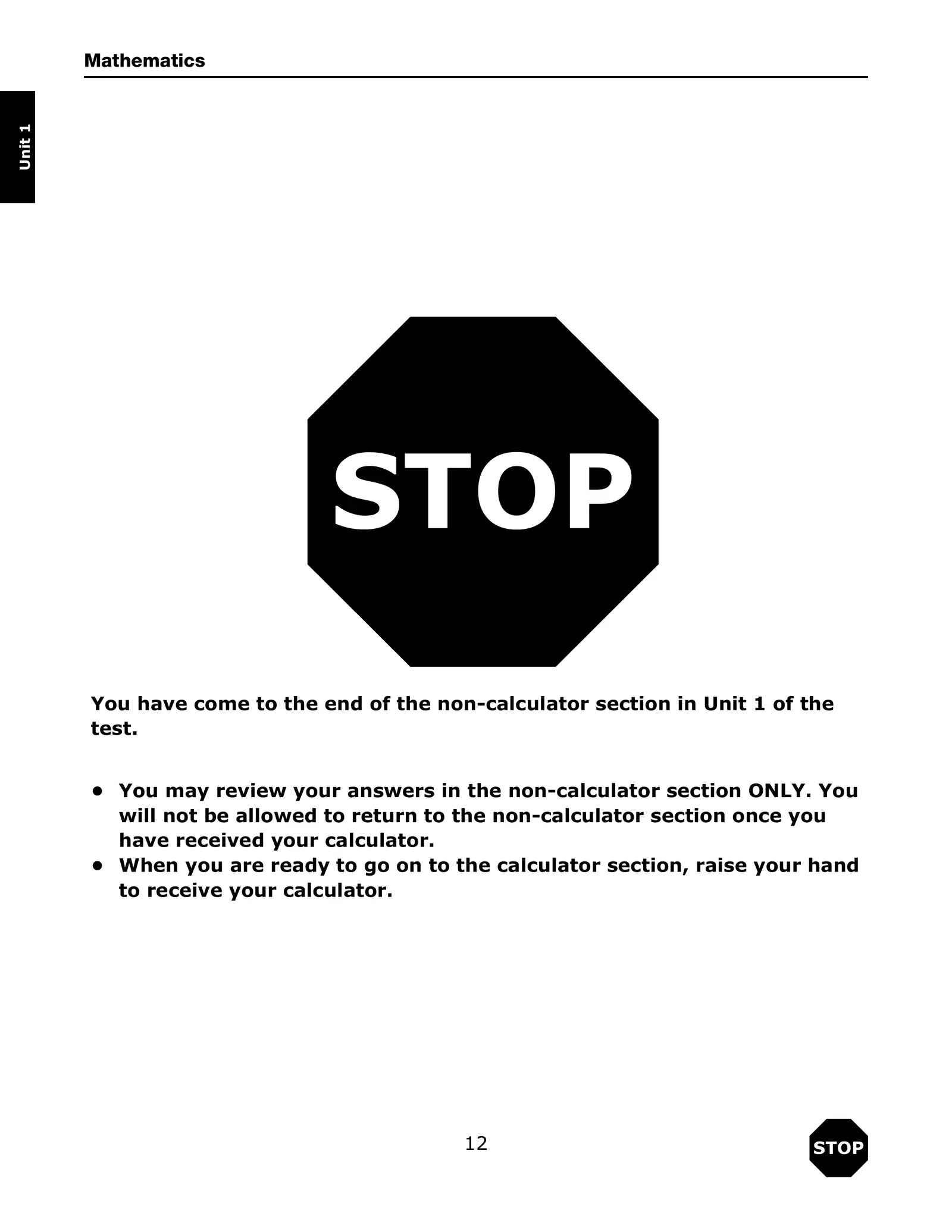Mathematics Unit 1 STOP You have come to the end of the non-calculator section in Unit 1 of the test. • You may review your answers in the non-calculator section ONLY. You will not be allowed to return to the non-calculator section once you have received your calculator. • When you are ready to go on to the calculator section, raise your hand to receive your calculator. 12 STOP